This book is archived and will be removed July 6, 2022. Please use the updated version.

Alternating-Current Circuits

# 103 Power in an AC Circuit

### Learning Objectives

By the end of the section, you will be able to:

• Describe how average power from an ac circuit can be written in terms of peak current and voltage and of rms current and voltage
• Determine the relationship between the phase angle of the current and voltage and the average power, known as the power factor

A circuit element dissipates or produces power according to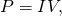where I is the current through the element and V is the voltage across it. Since the current and the voltage both depend on time in an ac circuit, the instantaneous power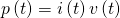is also time dependent. A plot of p(t) for various circuit elements is shown in (Figure). For a resistor, i(t) and v(t) are in phase and therefore always have the same sign (see (Figure)). For a capacitor or inductor, the relative signs of i(t) and v(t) vary over a cycle due to their phase differences (see (Figure) and (Figure)). Consequently, p(t) is positive at some times and negative at others, indicating that capacitive and inductive elements produce power at some instants and absorb it at others.

Graph of instantaneous power for various circuit elements. (a) For the resistor,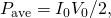whereas for (b) the capacitor and (c) the inductor,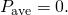(d) For the source,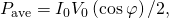which may be positive, negative, or zero, depending on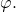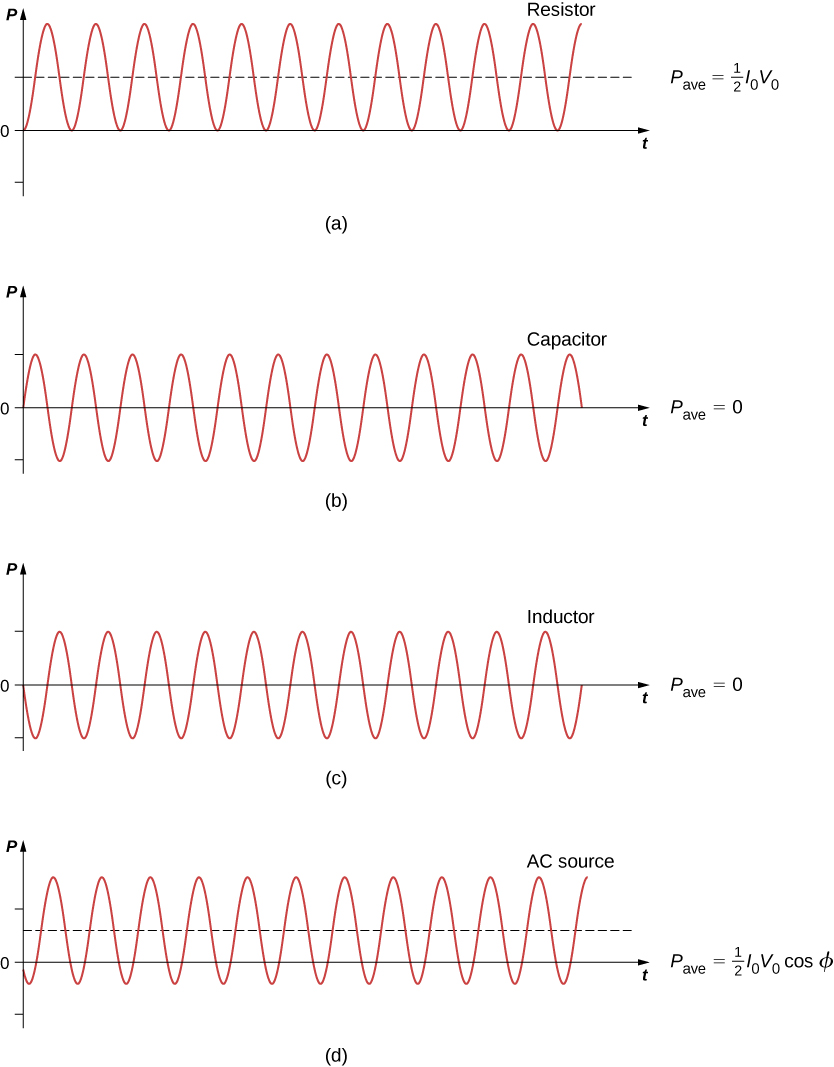Because instantaneous power varies in both magnitude and sign over a cycle, it seldom has any practical importance. What we’re almost always concerned with is the power averaged over time, which we refer to as the average power. It is defined by the time average of the instantaneous power over one cycle: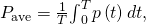where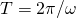is the period of the oscillations. With the substitutions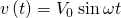and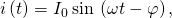this integral becomes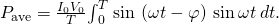Using the trigonometric relation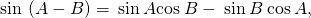we obtain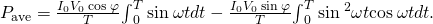Evaluation of these two integrals yields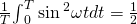and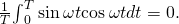Hence, the average power associated with a circuit element is given by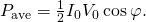In engineering applications,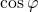is known as the power factor, which is the amount by which the power delivered in the circuit is less than the theoretical maximum of the circuit due to voltage and current being out of phase. For a resistor,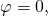so the average power dissipated is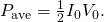A comparison of p(t) and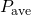is shown in (Figure)(d). To make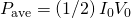look like its dc counterpart, we use the rms values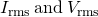of the current and the voltage. By definition, these are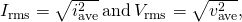where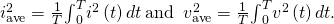With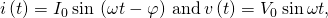we obtain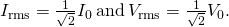We may then write for the average power dissipated by a resistor,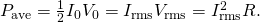This equation further emphasizes why the rms value is chosen in discussion rather than peak values. Both equations for average power are correct for (Figure), but the rms values in the formula give a cleaner representation, so the extra factor of 1/2 is not necessary.

Alternating voltages and currents are usually described in terms of their rms values. For example, the 110 V from a household outlet is an rms value. The amplitude of this source is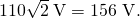Because most ac meters are calibrated in terms of rms values, a typical ac voltmeter placed across a household outlet will read 110 V.

For a capacitor and an inductor,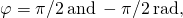respectively. Since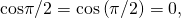we find from (Figure) that the average power dissipated by either of these elements isCapacitors and inductors absorb energy from the circuit during one half-cycle and then discharge it back to the circuit during the other half-cycle. This behavior is illustrated in the plots of (Figure), (b) and (c), which show p(t) oscillating sinusoidally about zero.

The phase angle for an ac generator may have any value. If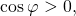the generator produces power; if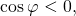it absorbs power. In terms of rms values, the average power of an ac generator is written as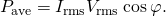For the generator in an RLC circuit,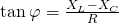and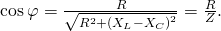Hence the average power of the generator is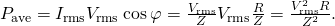This can also be written as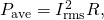which designates that the power produced by the generator is dissipated in the resistor. As we can see, Ohm’s law for the rms ac is found by dividing the rms voltage by the impedance.

Power Output of a Generator An ac generator whose emf is given by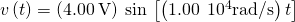is connected to an RLC circuit for which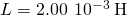,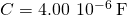, and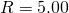. (a) What is the rms voltage across the generator? (b) What is the impedance of the circuit? (c) What is the average power output of the generator?

Strategy The rms voltage is the amplitude of the voltage times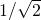. The impedance of the circuit involves the resistance and the reactances of the capacitor and the inductor. The average power is calculated by (Figure), or more specifically, the last part of the equation, because we have the impedance of the circuit Z, the rms voltage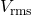, and the resistance R.

Solution

1. Since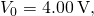the rms voltage across the generator is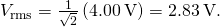2. The impedance of the circuit is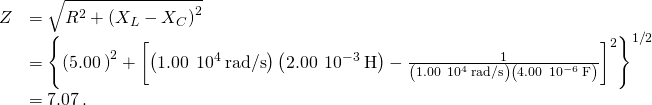3. From (Figure), the average power transferred to the circuit is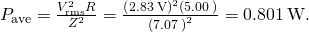Significance If the resistance is much larger than the reactance of the capacitor or inductor, the average power is a dc circuit equation of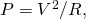where V replaces the rms voltage.

Check Your Understanding An ac voltmeter attached across the terminals of a 45-Hz ac generator reads 7.07 V. Write an expression for the emf of the generator.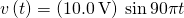Check Your Understanding Show that the rms voltages across a resistor, a capacitor, and an inductor in an ac circuit where the rms current is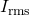are given by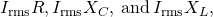respectively. Determine these values for the components of the RLC circuit of (Figure).

2.00 V; 10.01 V; 8.01 V

### Summary

• The average ac power is found by multiplying the rms values of current and voltage.
• Ohm’s law for the rms ac is found by dividing the rms voltage by the impedance.
• In an ac circuit, there is a phase angle between the source voltage and the current, which can be found by dividing the resistance by the impedance.
• The average power delivered to an RLC circuit is affected by the phase angle.
• The power factor ranges from –1 to 1.

### Conceptual Questions

For what value of the phase angle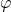between the voltage output of an ac source and the current is the average power output of the source a maximum?

Discuss the differences between average power and instantaneous power.

The instantaneous power is the power at a given instant. The average power is the power averaged over a cycle or number of cycles.

The average ac current delivered to a circuit is zero. Despite this, power is dissipated in the circuit. Explain.

Can the instantaneous power output of an ac source ever be negative? Can the average power output be negative?

The instantaneous power can be negative, but the power output can’t be negative.

The power rating of a resistor used in ac circuits refers to the maximum average power dissipated in the resistor. How does this compare with the maximum instantaneous power dissipated in the resistor?

### Problems

The emf of an ac source is given by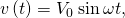where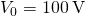and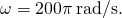Calculate the average power output of the source if it is connected across (a) a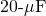capacitor, (b) a 20-mH inductor, and (c) a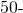resistor.

Calculate the rms currents for an ac source is given bywhereand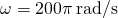when connected across (a) acapacitor, (b) a 20-mH inductor, and (c) aresistor.

a. 0.89 A; b. 5.6A; c. 1.4 A

A 40-mH inductor is connected to a 60-Hz AC source whose voltage amplitude is 50 V. If an AC voltmeter is placed across the inductor, what does it read?

For an RLC series circuit, the voltage amplitude and frequency of the source are 100 V and 500 Hz, respectively;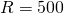; and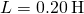. Find the average power dissipated in the resistor for the following values for the capacitance: (a)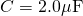and (b)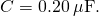a. 7.3 W; b. 6.3 W

An ac source of voltage amplitude 10 V delivers electric energy at a rate of 0.80 W when its current output is 2.5 A. What is the phase anglebetween the emf and the current?

An RLC series circuit has an impedance of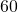and a power factor of 0.50, with the voltage lagging the current. (a) Should a capacitor or an inductor be placed in series with the elements to raise the power factor of the circuit? (b) What is the value of the reactance across the inductor that will raise the power factor to unity?

a. inductor; b.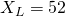### Glossary

average power
time average of the instantaneous power over one cycle
power factor
amount by which the power delivered in the circuit is less than the theoretical maximum of the circuit due to voltage and current being out of phase